# 指数与对数

## 什么是指数？一个数的指数代表把多少个 这个数 乘在一起。 例子： 23 = 2 × 2 × 2 = 8 （3个 2 乘在一起得到 8）

## 什么是对数？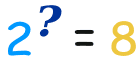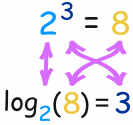• 指数用 2 和 3 来得到 8 （2乘3次为8）
• 对数用 2 和 8 来得到 3 （2 成为 8，当把3个2乘在一起时）（去这里看看指数、根和对数的关系。）

## 一起用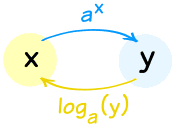• ax，然后取对数，得回 x: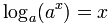• 取对数，然后取 ax，得回 x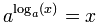• 向上走，然后向下走，你回到原处：向下(向上p(x)) = x
• 向下走，然后向上走，你回到原处：向上(向下(x)) = x

（反过来也一样）

### 举例： log3(x) = 5，x 是什么？

 开始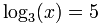我们想"还原"对数以得到 "x =" 每边都用指数函数：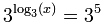我们知道，所以： x = 35 答案： x = 243

### 例子：y=log4(1/4)，求 y

 开始每边都用指数函数：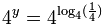简化： 4y = 1/4 小窍门：1/4 = 4-1 所以： 4y = 4-1 故此： y = -1

## 对数的特性

loga( m × n ) = logam + logan

"乘的对数是对数的和"

### 为什么是这样？看附注。

 loga(m × n) = logam + logan 乘的对数是对数的和 loga(m/n) = logam - logan 除乘的对数是对数的差 loga(1/n) = -logan 这是以上"除"特性的结果，因为 loga(1) = 0 loga(mr) = r ( logam ) m的r次幂 的对数 是 r 和 m的对数 的积历史： 以前没有计算器时，对数非常有用……例如，要乘两个很大的数，你可以用对数来把乘变为加（容易得多！）

### 例子：简化 loga( (x2+1)4√x )

 开始： loga( (x2+1)4√x ) 用 loga(mn) = logam + logan： loga( (x2+1)4 ) + loga( √x ) 用 loga(mr) = r ( logam ) : 4 loga(x2+1) + loga( √x ) 同时 √x = x½ : 4 loga(x2+1) + loga( x½ ) 再用 loga(mr) = r ( logam ) ： 4 loga(x2+1) + ½ loga(x)

### 例子：把loga(5) + loga(x) − loga(2) 变成一个对数：

 开始： loga(5) + loga(x) − loga(2) 用 loga(mn) = logam + logan : loga(5x) − loga(2) 用 loga(m/n) = logam − logan : loga(5x/2)

## 自然对数和自然指数函数

• 自然对数 loge(x)
通常写为 ln(x)
• 自然指数函数 ex

ln(ex) = x

e(ln x) = x

 自然对数 自然指数函数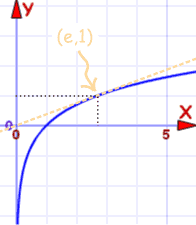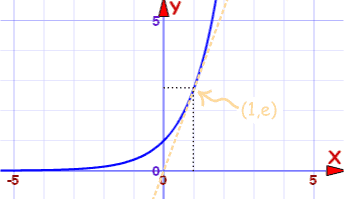f(x) = ln(x)的图 f(x) = ex的图 穿过 (1,0) 和 (e,1) 穿过 (0,1) 和 (1,e)在计算器上，自然对数是 "ln" 键。

## 常用对数

• 常用对数 log10(x)，有时写为 log(x)在计算器上，常用对数是 "log" 键。 它的有用之处是告诉你数在十进制里 "有多大"（你要乘几个10）。

### 例子：计算 log10 100

10 × 10 = 100，所以2个 10乘在一起的积是 100：

log10 100 = 2

### 例子：计算 log10 369

log10 369 = 2.567……

## 改变底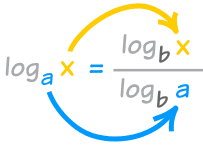"x 增大，a 减小"

loga x = logb x / logb a

loga x = 1 / logx a

### 例子：计算 1 / log8 2

1 / log8 2 = log2 8

2 × 2 × 2 = 8，所以3个 2乘在一起的积是 8：

1 / log8 2 = log2 8 = 3

loga x = ln x / ln a

### 例子：计算 log4 22我的计算器没有 "log4" 键…… ……但它有 "ln" 键。我们来用它：

log4 22 = ln 22 / ln 4 = 3.09.../1.39... = 2.23 （保留三位小数）

### 例子：计算 log5 125

log5 125 = ln 125 / ln 5 = 4.83.../1.61... = 3 （绝对精确）

M = log10 A + B

B 是距离校正系数

pH = −log10 [H+]

## 更多例子

### 例子：解 2 log8 x = log8 16

 开始： 2 log8 x = log8 16 把 "2" 带进对数：: log8 x2 = log8 16 拿走对数（对数的底相同）： x2 = 16 解： x = −4 or +4

### 例子：解 e−w = e2w+6

 开始： e−w = e2w+6 每边取 ln： ln(e−w) = ln(e2w+6) ln(ew)=w： −w = 2w+6 简化： −3w = 6 解： w = 6/−3 = −2

### 附注：为什么 log(m × n) = log(m) + log(n)？

 首先把 m 和 n 变成 "对数的指数"：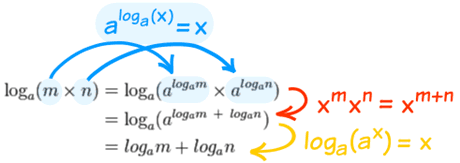然后用指数定律 最后把指数还原。